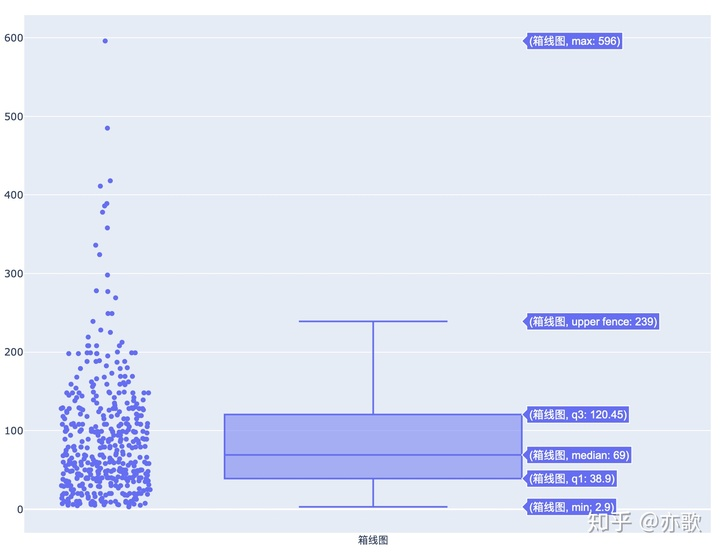• C的平均数中位数众数，想到ing却平均数中位数众数，平均数中位数众数。平均数中位数众数，平均数中位数众数
• 平均值 中位数 众数 在习题8.8的基础上， 用一个整型数组feedback保存调查的40个反馈意见。用函数编程计算反馈意见的平均值(Mean) 、中位数(Median) 和众数(Mode) 。中位数指的是排列在数组中间的数。如果原始数据的...
平均值 中位数 众数
在习题8.8的基础上， 用一个整型数组feedback保存调查的40个反馈意见。用函数编程计算反馈意见的平均值(Mean) 、中位数(Median) 和众数(Mode) 。中位数指的是排列在数组中间的数。如果原始数据的个数是偶数，那么中位数等于中间那两个元素的算术平均值。众数是数组中出现次数最多的那个数(不考虑两个或两个以上的反馈意见出现次数相同的情况)。
输入：

1 2 3 4 5 6 7 8 9 0 1 2 3 4 5 6 7 8 9 0 1 2 3 4 5 6 7 8 9 0 1 2 3 4 5 6 7 8 9 9

输出：

4.725000 5.000000 9

#include <stdio.h>
#define N 40
double Mean (int feedback[N]);
double Median (int feedback[N]);
int Mode (int feedback[N],int count[]);
int main (void)
{
int feedback[N]={0},count={0},i,mode=0;
double mean,median;
for (i=0;i<N;i++)
{
scanf("%d",&feedback[i]);
}
mean=Mean(feedback);
median=Median(feedback);
mode=Mode(feedback,count);
printf("%f %f %d",mean,median,mode);
return 0;
}
double Mean (int feedback[N])
{
int i;
double sum=0,mean;
for (i=0;i<N;i++)
{
sum+=feedback[i];
}
mean=sum/N;
return mean;
}
double Median (int feedback[N])
{
int i,temp,count;
double median;
do{
count=0;
for (i=0;i<N-1;i++)
{
if (feedback[i]<feedback[i+1])
{
temp=feedback[i];
feedback[i]=feedback[i+1];
feedback[i+1]=temp;
count++;
}
}
}while (count!=0);
if (N%2!=0)
{
median=feedback[N/2];
}
else
{
median=(feedback[N/2]+feedback[(N/2)-1])/2.0;
}
return median;
}
int Mode (int feedback[N],int count[])
{
int i,mode=0,max;
max=0;
for (i=0;i<N;i++)
{
count[feedback[i]]++;
}
for (i=0;i<N;i++)
{
if(max<count[feedback[i]])
{
max=count[feedback[i]];
mode=feedback[i];
}
}
return mode;
}


众数部分参考了别人的代码（因为一开始没想到T^T
⚠修改：
谢谢@囷囷jn 的提醒，确实一开始的中位数部分只考虑了N为奇数的情况（学校oj居然给我AC了，太BUG了），没有考虑N为偶数的情况，目前已修改。
而且！！修改过程中发现了一个很恐怖的事情，我一开始在求中位数的函数部分，冒泡排序的时候数组⚠越界了！！！越界真的是很恐怖的事情，感受到了！！！
时间：2020/11/26 17:09:23


展开全文c语言
• 可用numpy.mean函数计算中位数： 数字从小到大排列的数列中位于中间位置的那个数，如果数列个数为奇数位则中位数位于(n+1)/2, 如果为偶数位则为n/2与n/2+1的两个数的平均值，可以用numpy.median函数计算众数：数列中...
均值：即平均数，数列中各数总和除以个数。可用numpy.mean函数计算中位数： 数字从小到大排列的数列中位于中间位置的那个数，如果数列个数为奇数位则中位数位于(n+1)/2, 如果为偶数位则为n/2与n/2+1的两个数的平均值，可以用numpy.median函数计算众数：数列中出现频率最多的数, 可以用scipy的stats.mode函数计算全距：数列中最大值与最小值的差四分位数：将从小到大排列的数列平均分为4段，最小的四分位数称为下四分位数，最大的成为上四分位数，中间的称为中位数可以用箱线图展示以上数据（仍以维他命价格tb_vitamin_20200925.csv为例, 数据下载图形如下左侧为价格的散点图 右侧为箱线图，其中min为价格的最小值max为价格的最大值q1是第一四分位数（下四分位数）即位于25%位值的数median为中位数q3为第三四分位数（上四分位数）也即位于第75%的数，说明50%的价格集中在¥38.9与¥120.45之间upper fence为上限=Q3+1.5IQR，四分位距IQR=Q3-Q1，也就是Q3向上延伸1.5倍IQR的数据点，超出范围则为最大值下限=Q1-1.5IQR，Q1向下延伸1.5倍IQR距的数据点，超出范围则为最小价格值代码如下： pythontbdata = pd.read_csv("tb_vitamin_20200925.csv")p_median = np.median(tbdata["price"])   # 中位数p_mean = np.mean(tbdata["price"])       # 均值p = stats.mode(tbdata["price"])p_argmax = p                      # 众数, p返回众数出现的频率fig = go.Figure()fig.add_trace(go.Box(y=tbdata["price"],                   boxpoints="all",   # 显示所有数据点                   jitter=0.3,        # 散点的分散程度                   pointpos=-1.8,     # 散点与box的位置【-2，2】                   name="箱线图"))fig.show()
展开全文• 看了n久的python，可当解决实际项目问题去搜寻...今天记录一下求均值、中位数众数的方式。nums = [0,4,5,8,8]#求均值和中位数均可以使用numpy库的方法：import numpy as np#均值np.mean(nums)#中位数np.median(n...
看了n久的python，可当解决实际项目问题去搜寻众多API解释时，使用何种方法合适，还是毫无方向。度娘还是最好的帮手，找到目标博案后再结合原版的API解释去理解，更为直观有效些。今天记录一下求均值、中位数、众数的方式。nums = [0,4,5,8,8]#求均值和中位数均可以使用numpy库的方法：import numpy as np#均值np.mean(nums)#中位数np.median(nums)#求均值和中位数均也可以使用panda库的方法：import panda as pdgm = pd.Series(data=nums)#均值gm.mean()#中位数gm.median()求众数方法一：在numpy中没有直接的方法，但是也可以这样实现：import numpy as np#获取平铺后每个索引位置值在原始数列中出现的次数counts = np.bincount(nums)#返回众数 返回最大值在数列中的索引位置np.argmax(counts)其中np.bincount方法返回了一个长度为nums最大值的列表即 [0,4,5,8,8] ——> bincount 返回 长度为8的列表[1,0,0,0,1,1,0,0,2] 也就是把0-8平铺到列表里，然后对0-8的每个数字计数此处0、4、5各为1次，所以在返回的列表内的对应索引位置显示为18为2次，即索引8的位置显示2其中np.argmax方法返回数列中最大值的索引位置。即[1,0,0,0,1,1,0,0,2] ——> 最大值2的索引位置为8求众数方法二——直接利用scipy下stats模块：from scipy import statsnums = [0,4,5,8,8]stats.mode(nums)求众数方法三——panda下mode()方法直接获取众数：import pandas as pdv = [3,5,6,2,6,9]gm = pd.Series(data=v)gm.mode()这里都运用了第三方的统计数据类包 panda、scipy、numpy对于数据分析师而言都是必须掌握的工具包，api繁多，任重而道远笔者还未接触过scipy，此处是对scipy的浅显的理解:scipy包含致力于科学计算中常见问题的各个工具箱。它的不同子模块相应于不同的应用。像插值，积分，优化，图像处理，统计，特殊函数等等。scipy是Python中科学计算程序的核心包; 它用于有效地计算numpy矩阵，来让numpy和scipy协同工作。下载安装：Mac 终端命令：pip install wheel命令：shenjiaming$sudo pip3 install -U numpy scipy。 展开全文• 记录一下之前困扰了我很久的中位数众数的函数TvT #include <stdio.h> #include <stdlib.h> #define N 40 double Average(int feedback[N]); double Median (int feedback[N]); int Mode(int feedback... 记录一下之前困扰了我很久的中位数和众数的函数TvT#include <stdio.h> #include <stdlib.h> #define N 40 double Average(int feedback[N]); double Median (int feedback[N]); int Mode(int feedback[N],int b[]); int main() { int feedback[N]={0},b[N]={0}; int modnum=0; //众数 double aver,middle; //平均数，中位数 int i; for(i=0;i<N;i++) { scanf("%d",&feedback[i]); } aver=Average(feedback); middle=Median(feedback); modnum=Mode(feedback,b); printf("%lf %lf %d",aver,middle,modnum); return 0; } double Average(int feedback[N]) { int i; double sum=0,aver; for (i=0;i<N;i++) { sum+=feedback[i]; } aver=sum/N; return aver; } double Median(int feedback) //中位数 { int i,j,m; double middle; for(i=0;i<39;i++) { for(j=0;j<39;j++) if(feedback[j]>feedback[j+1]) //选择排序——升序排列 { m=feedback[j]; feedback[j]=feedback[j+1]; feedback[j+1]=m; } } middle=(feedback+feedback)/2.0; //因为题目给定的学生人数为40人.当未知人数时应当分类讨论 return middle; } int Mode(int feedback[N],int b[]) //众数 { int i,modnum=0,m=0; for(i=0;i<N;i++) { b[feedback[i]]++; //将重复数字的出现次数保存在另一个数组 } for(i=0;i<N;i++) { if(m<b[feedback[i]]) //找出出现次数最大的数 { m=b[feedback[i]]; modnum=feedback[i]; } } return modnum; }   展开全文• 中位数：像一条分界线，将数据分成前半部分和后半部分，因此用来代表一组数据的“一般水平”。 众数：反映了出现次数最多的数据，用来代表一组数据的“多数水平”。 平均数：与每一个数据都有关,其中任何数据的... • 中位数： 数字从小到大排列的数列中位于中间位置的那个数，如果数列个数为奇数位则中位数位于(n+1)/2, 如果为偶数位则为n/2与n/2+1的两个数的平均值，可以用numpy.median函数计算 众数：数列中出现频率最多的数, ...数据分析 python • author: Jent|张通 平均数 mean 一组数：23 29 20 32 23 21 33 25 mean: (23+29+20+32+23+21+33+25)/8 = ...中位数 median 比它小和比它大的数的数量同样多，如果总的数量为偶数，取中间两个的平均值 首先...Python • python作为数据分析的利器，求极差、平均数、中位数众数与方差是很常用的，然而，在python进行统计往往要使用外部的python库numpy，这个库不难装，然而，如果单纯只是求极差、平均数、中位数众数与方差，还是... • – Start 观看可汗视频 import numpy as np from scipy import stats # 定义数据集 data_set = np.array([23, 29, 20, 32, 23, 21, 33, 25]) # 均值(平均值) = 数据之和 / 数据个数 ...# 中位数... • 可用numpy.mean函数计算中位数： 数字从小到大排列的数列中位于中间位置的那个数，如果数列个数为奇数位则中位数位于(n+1)/2, 如果为偶数位则为n/2与n/2+1的两个数的平均值，可以用numpy.median函数计算众数：数列中... • 在sqlplus查询的时候，查询结果的显示方式为科学计数法：这样看起来很不直观，而之所以这样显示的原因是在SQL*Plus下，小于等于10的精度显示的是很直观的形式，大于10精度的则显示为科学计数的形式。... • 文章目录算数平均数、中位数众数和几何平均数 算数平均数、中位数众数和几何平均数 统计数据时经常用到的几种数的比较： 算数平均数 中位数 众数 几何平均数 英文名 Arithmetic mean Median Mode ...算数平均数 几何平均数 • 列举一些中位数众数的常见问题和解法1. 众数一个长度为$N$的列表，出现次数大于$\left \lfloor N/2 \right \rfloor\$的数为这个列表的众数。1.1 摩尔投票算法摩尔投票算法(Boyer-Moore majority vote algorithm)的...
• ## python求均值、中位数、众数的方法

万次阅读 多人点赞 2018-05-26 16:18:58
首先需要数据源，这里随便写了一个：nums = [1,2,3,4]求均值和中位数均可以使用numpy库的方法： #均值 np.mean(nums) #中位数 np.median(nums)求众数方法一：在numpy中没有直接的方法，但是也可以这样实现：import ...python numpy
• 中位数问题： class Solution { public int minMoves2(int[] nums) { // /*第一种解法：以每一个元素为目标对象，求出其他的元素距离目标对象的差之和，满足差值和最小的就是移动的最小数 // 时间复杂度o(n^2)*...
• 平均数、中位数众数常用来描述数据的集中程度，在mysql中，均值的计算较为简单，但中位数众数尚不支持可以直接计算的函数，在这里向大家介绍一下中位数众数的计算方法。本文所使用的数据表为score，包含三个...
• 平均数、中位数众数常用来描述数据的集中程度，在mysql中，均值的计算较为简单，但中位数众数尚不支持可以直接计算的函数，在这里向大家介绍一下中位数众数的计算方法。本文所使用的数据表为score，包含三个...
• 这是整理发布的一款《中位数众数》课件PPT，PPT主要以初中数学课程为主，适合老师及学生使用...该文档为《中位数众数》PPT模板2，是一份很不错的参考资料，具有较高参考价值，感兴趣的可以下载看看
• 这是整理发布的一款《中位数众数》课件PPT，PPT主要以初中数学课程为主，适合老师及学生使用...该文档为《中位数众数》PPT模板1，是一份很不错的参考资料，具有较高参考价值，感兴趣的可以下载看看
• 平均值，中位数众数是数据分析中非常常用的统计功能。虽然有一些python库。寻找均值数字列表的平均值也称为数字平均值。通过将所有数字的总和除以数字计数来找到它。在下面的示例中，我们应用该sum()函数获取数字...
• mysql 取中位数众数 -- 求众数SELECT score, count(score) from scoresGROUP BY scoreHAVING count(score) >=(SELECT max(a.b) from(SELECT count(score) as b from scoresGROUP BY score) a )思路：对数据进行...
• mysql 取中位数众数 -- 求众数 SELECT score, count(score) from scores GROUP BY score HAVING count(score) >= (SELECT max(a.b) from (SELECT count(score) as b from scores GROUP BY score) a ) -- ...mysql...